by Vicky Avison

### Categories

If you’ve done many joins in Spark, you’ve probably encountered the dreaded Data Skew at some point. The initial elation at how quickly Spark is ploughing through your tasks (“Wow, Spark is so fast!”) is later followed by dismay when you realise it’s been stuck on 199/200 tasks complete for the last 5 hours.

In this post I’ll aim to explain what a data skew is, how to identify when you have one, and talk about one of the tactics we’ve used to tackle our own data skews (or ‘Fiestas’, as we like to call them)

## What is a data skew?

In order to perform a join, Spark needs to co-locate rows with the same join key. To do this, it assigns a partition id to each row based upon the hash of its key (what we are joining on). In an ideal world, our join keys would be nicely distributed, and each partition would get an even number of records to process. However, real business data does not often equal an ideal world, and we end up with skews.

Let’s consider a simple example which bears a resemblance to something we recently had to deal with. The problem:

• We have some cars (registration, make, model, engine size) (let’s call this t1)
• We have a large dataset containing a lot more cars and their sale prices (let’s call this t2)
• We say two vehicles are ‘similar’ if they have the same make and model and engine sizes within 0.1 litres of each other (this is a much simplified version of the real ‘similar vehicles’ definition our Data Scientists came up with)
• For each car in t1, we want to calculate the average price of ‘similar’ vehicles in t2

So far, so simple.

t1.join(t2, Seq("make", "model"))
.filter(abs(t2("engine_size") - t1("engine_size")) <= BigDecimal("0.1"))
.groupBy("registration")
.agg(avg("sale_price").as("average_price")


The problem here is that the make-model combination is definitely not evenly distributed. There are loads of Ford Fiestas in the wild, and Spark was throwing them all into the same partition. Skew hell!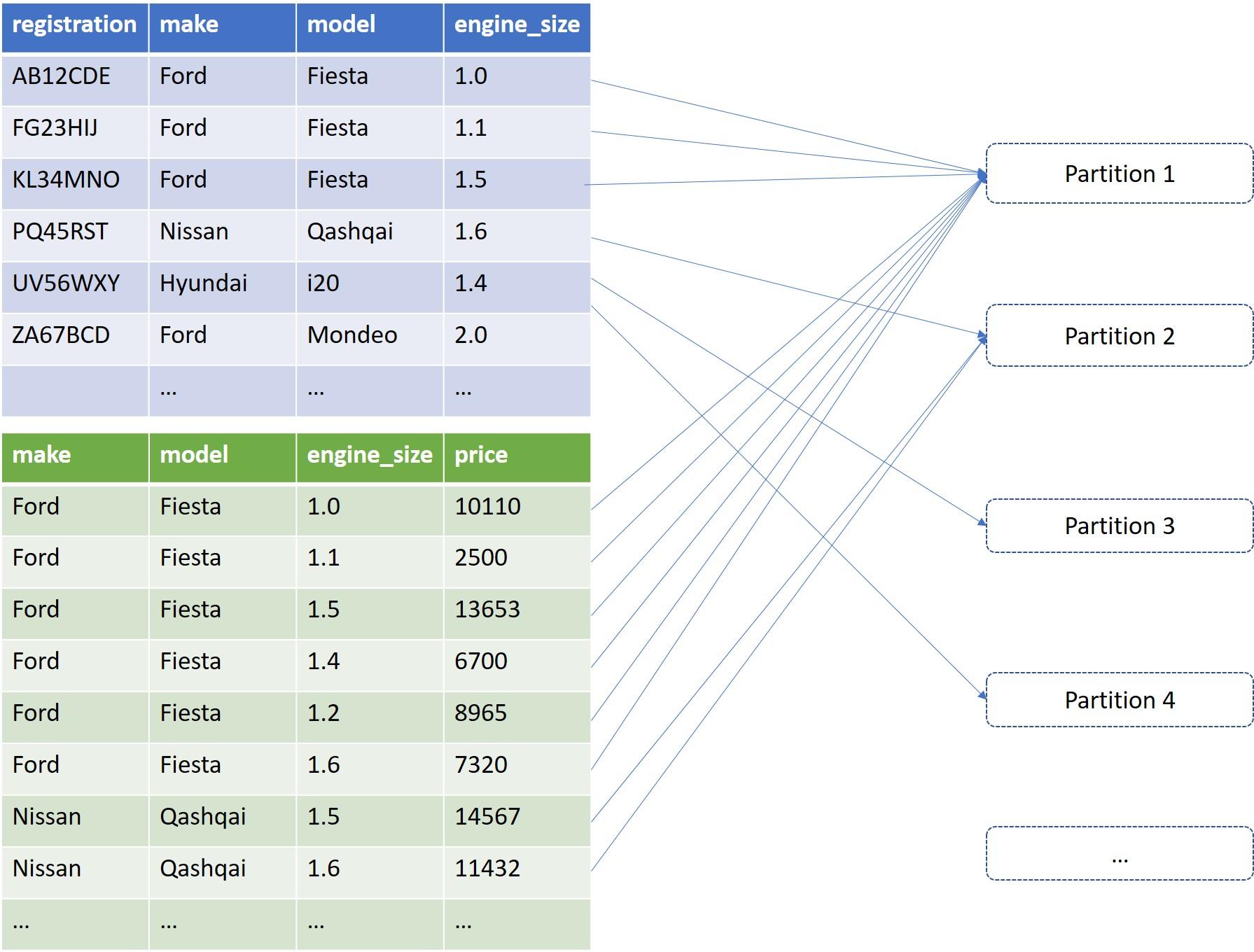## How to tell if your join is skewing

If your join has been running for a long time, and you don’t think it should be, then it could well be skewing. You can get a lot more information by looking in the Spark UI.

Let’s work through an example of a join which will skew. First let’s generate some skewed data to work with. The following will generate t1 and t2 as described above, where Ford Fiestas account for roughly 50% of all vehicles.

import scala.util.Random
import scala.math.BigDecimal

case class MakeModel(make: String, model: String)

case class T1(registration: String, make: String, model: String, engine_size: BigDecimal)

case class T2(make: String, model: String, engine_size: BigDecimal, sale_price: Double)

val makeModelSet: Seq[MakeModel] = Seq(
MakeModel("FORD", "FIESTA")
, MakeModel("NISSAN", "QASHQAI")
, MakeModel("HYUNDAI", "I20")
, MakeModel("SUZUKI", "SWIFT")
, MakeModel("MERCEDED_BENZ", "E CLASS")
, MakeModel("VAUCHALL", "CORSA")
, MakeModel("FIAT", "500")
, MakeModel("SKODA", "OCTAVIA")
, MakeModel("KIA", "RIO")
)

def randomMakeModel(): MakeModel = {
val makeModelIndex = if (Random.nextBoolean()) 0 else Random.nextInt(makeModelSet.size)
makeModelSet(makeModelIndex)
}

def randomEngineSize() = BigDecimal(s"1.${Random.nextInt(9)}") def randomRegistration(): String = s"${Random.alphanumeric.take(7).mkString("")}"

def randomPrice() = 500 + Random.nextInt(5000)

def randomT1(): T1 = {
val makeModel = randomMakeModel()
T1(randomRegistration(), makeModel.make, makeModel.model, randomEngineSize())
}

def randomT2(): T2 = {
val makeModel = randomMakeModel()
T2(makeModel.make, makeModel.model, randomEngineSize(), randomPrice())
}

val t1 = Seq.fill(10000)(randomT1()).toDS()

val t2 = Seq.fill(100000)(randomT2()).toDS()


Now let’s run our join and take a look in the Spark UI to see what’s happening

//Because our datasets are small, Spark will try and do a broadcast join. In order to see our skew happening, we need to suppress this behaviour

t1.join(t2, Seq("make", "model"))
.filter(abs(t2("engine_size") - t1("engine_size")) <= BigDecimal("0.1"))
.groupBy("registration")
.agg(avg("sale_price").as("average_price")).collect()


If we look at our job in the Spark UI, we can see that the stage for the join finishes 199/200 tasks very quickly, and then spends a significant amount of time on the final remaining task.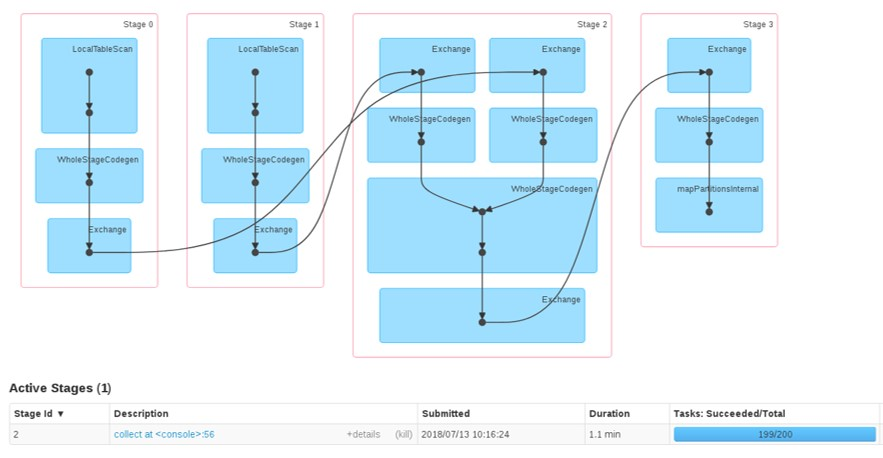If we look at the stage detail and order the tasks by duration descending, we can see our long-running task took almost 40 times longer than all of the others.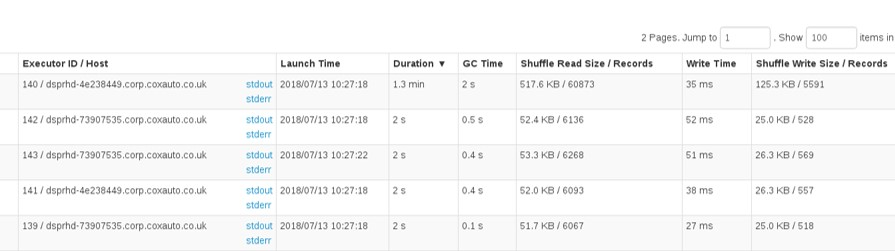On this volume of data it’s not too much of an issue - do we really care about that extra minute? But when our data volumes get large, this differential can turn into hours. If you’re waiting over 3 hours for one task to complete (as we were) then you really need to do something about it. Fortunately, there are some techniques we can use.

## Tackling the skew

I’m going to look at a couple of skew-fighting tactics in this two-part blog. The first is dependent on the scenario and what you have in the data but works really well. The second (which I will discuss in part 2) is a more general approach which should work on any problem.

### Tactic 1: is there anything in your data you can add to the join key?

What we really want to do is to break that big Ford Fiesta partition into smaller partitions, thereby distributing our processing better.

Looking again at our definition of ‘similar’ vehicles, in addition to the requirement that the make and model be the same, we also stipulate that the engine size can only vary by 0.1 litres. To achieve this we added a filter(abs(t2("engine_size") - t1("engine_size")) <= BigDecimal("0.1")) after the join. Could we have added this inequality into the join condition and thereby made our partitions smaller?

Yes, we could have added the inequality into the join condition, but it would have made no difference to the execution plan. Spark can only partition on equality join conditions. This makes sense, as it works out the partition number by performing a hash on the join key - how would this work with a range?

However, there is a way we can get engine size into the join key, and it’s due to an important quality of the data in this column: it’s discrete - it only varies in increments of 0.1.

How does this help us?

For each record in t1 we can add two more records, with engine sizes +/- 0.1 of the original. Then we can include engine size in the join condition!

What?

Take a look at the following, where all the records are going into the same partition (as per the original implementation).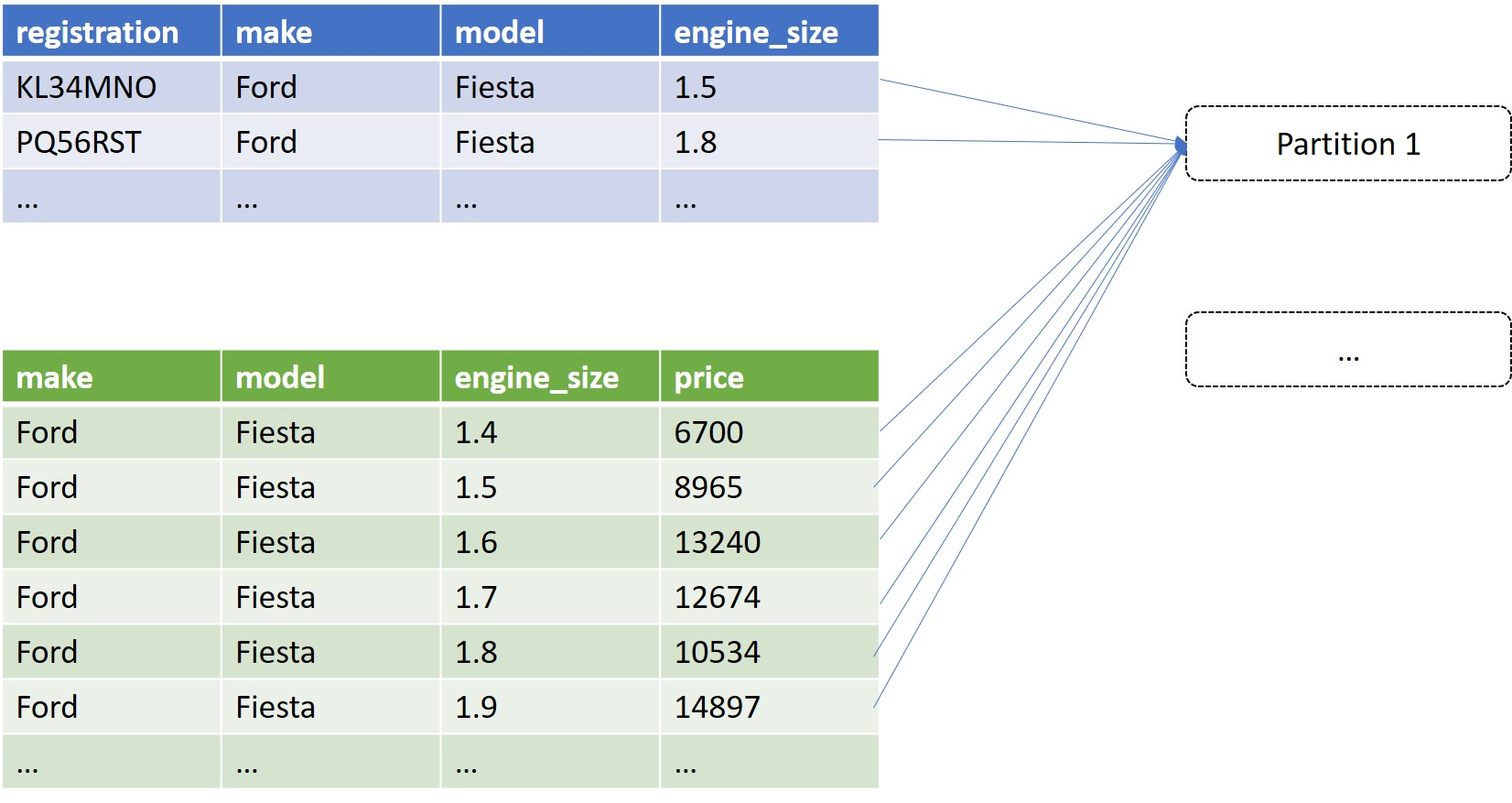If we take t1, and add two rows for each original row, with engine sizes 0.1 either side, we end up with three times as much data for t1 which you may think seems like a bad thing (surely just adding more data will make it slower?) However, we can now include engine_size in our join_condition to achieve the desired result (every original record from t1 will be joined to every record from t2 with the same make, model, and engine size +/- 0.1). The result is a much more even partition distribution.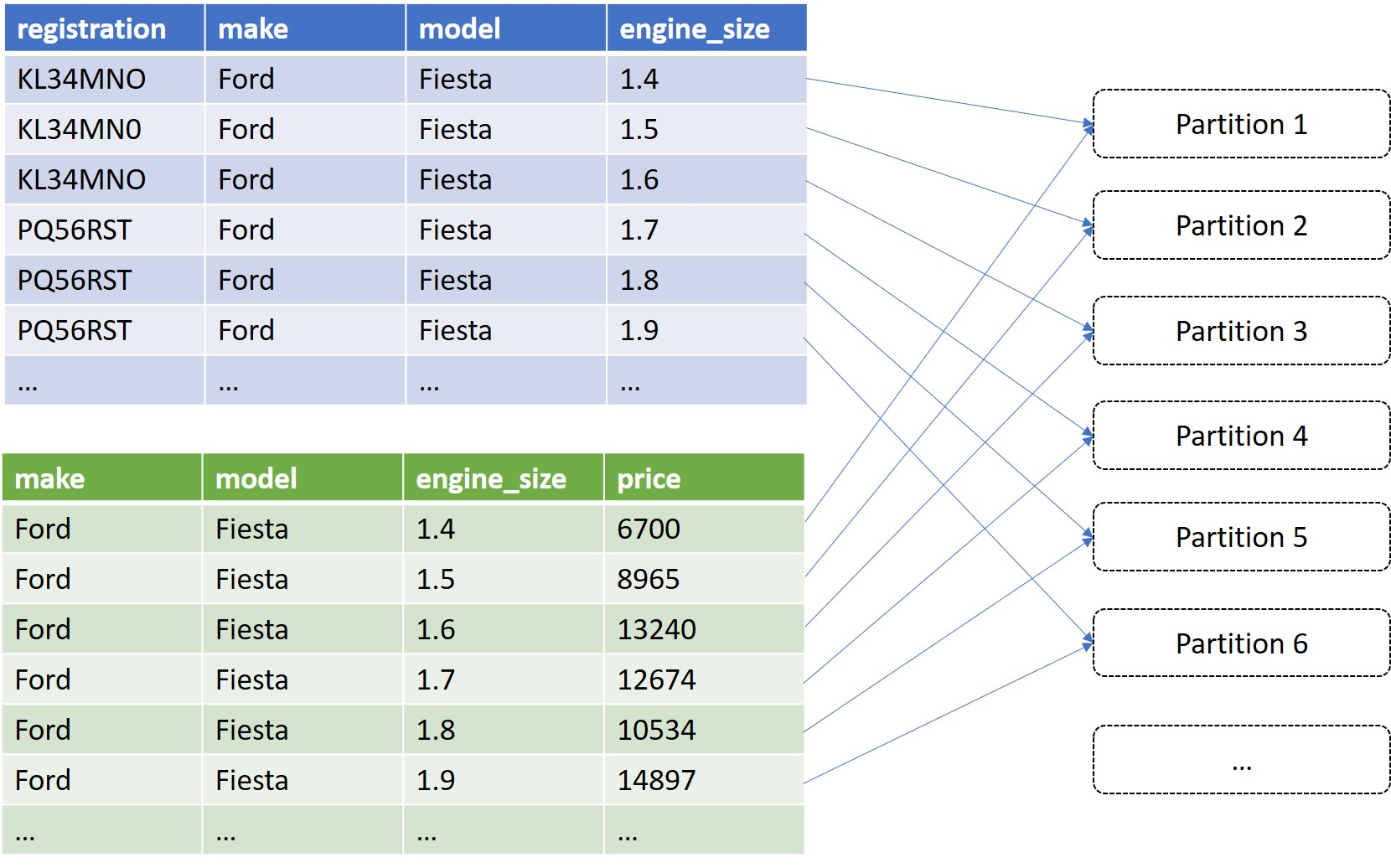How do we do this in Spark? Fortunately, there’s a very useful method called explode.

//Because our datasets are small, Spark will try and do a broadcast join. In order to see our skew happening, we need to suppress this behaviour

t1.withColumn("engine_size", explode(array($"engine_size" - BigDecimal("0.1"),$"engine_size", \$"engine_size" + BigDecimal("0.1"))))
.join(t2, Seq("make", "model", "engine_size"))
.groupBy("registration")
.agg(avg("sale_price").as("average_price"))
.collect()


If we look in the Spark UI, we can see that our processing is a lot more distributed - there doesn’t seem to be any skew at all now!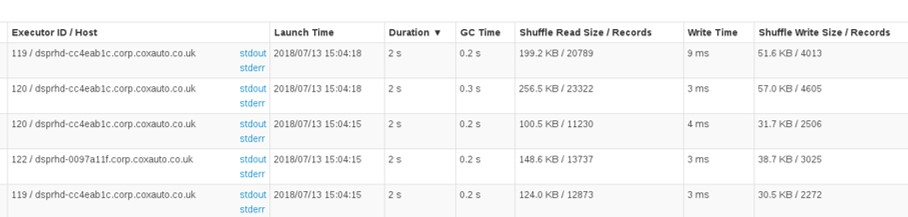Hopefully that’s demonstrated how paying attention to the Spark UI, and making some very small changes to your code, can literally save hours of job execution time.

Stay tuned for part 2 of this blog, where I’ll generalise the above strategy so you can use it in any skew situation.